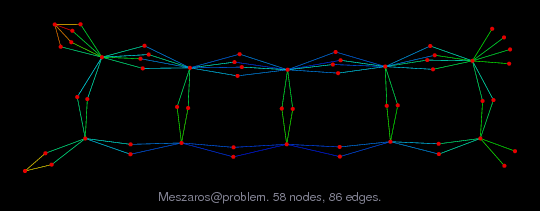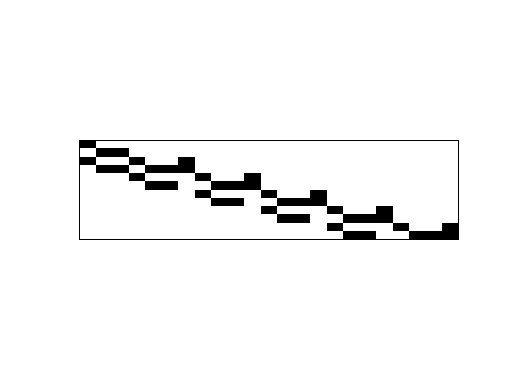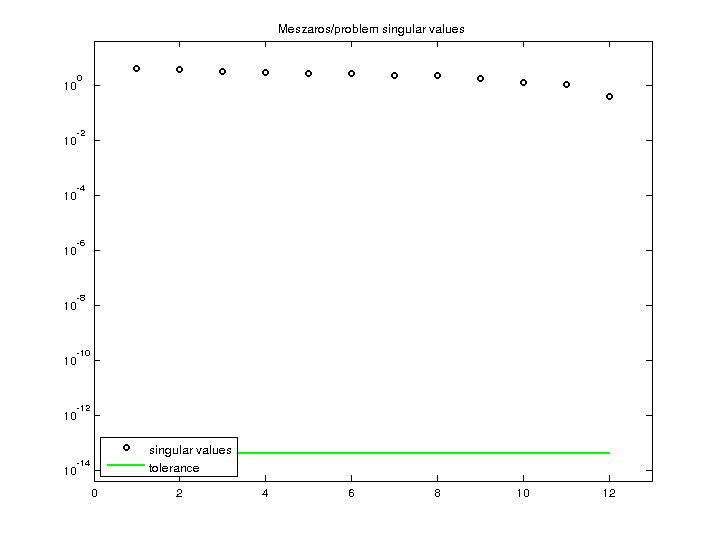Matrix: Meszaros/problem

Description: linear programming problem, C. Meszaros test set(bipartite graph drawing)• Matrix group: Meszaros
• download as a MATLAB mat-file, file size: 933 bytes. Use UFget(1755) or UFget('Meszaros/problem') in MATLAB.

 Matrix properties number of rows 12 number of columns 46 nonzeros 86 structural full rank? yes structural rank 12 # of blocks from dmperm 1 # strongly connected comp. 1 explicit zero entries 0 nonzero pattern symmetry 0% numeric value symmetry 0% type integer structure rectangular Cholesky candidate? no positive definite? no

 author editor C. Meszaros date 2004 kind linear programming problem 2D/3D problem? no

 Additional fields size and type b full 12-by-1 c full 46-by-1 lo full 46-by-1 hi full 46-by-1 z0 full 1-by-1

Notes:

```http://www.sztaki.hu/~meszaros/public_ftp/lptestset
Converted to standard form via Resende and Veiga's mpsrd:
minimize c'*x, subject to A*x=b and lo <= x <= hi
```

 Ordering statistics: result nnz(V) for QR, upper bound nnz(L) for LU, with COLAMD 213 nnz(R) for QR, upper bound nnz(U) for LU, with COLAMD 31

 SVD-based statistics: norm(A) 4.18872 min(svd(A)) 0.415481 cond(A) 10.0816 rank(A) 12 sprank(A)-rank(A) 0 null space dimension 0 full numerical rank? yes

 singular values (MAT file): click here SVD method used: s = svd (full (R)) ; where [~,R,E] = spqr (A') with droptol of zero status: ok